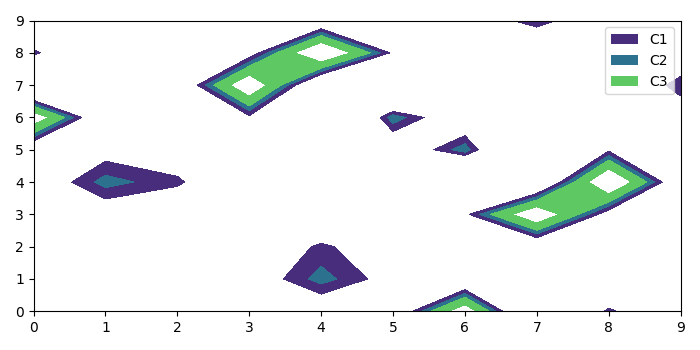# How do you create a legend for a contour plot in Matplotlib?

To create a legend for a contour plot in matplotlib, we can take the following steps−

• Create x, y and z data points to plot the contour function.
• To create a 3D filled contour plot, we can use contourf() method with x, y, z and different levels.
• Make a list of rectangle with the returned contour signature collection and set face color
• Now, place the legend in the plot using proxy (of step 3) and different labels.
• To display the figure, use show() method.

## Example

import numpy as np
from matplotlib import pyplot as plt
plt.rcParams["figure.figsize"] = [7.00, 3.50]
plt.rcParams["figure.autolayout"] = True
x, y = np.meshgrid(np.arange(10), np.arange(10))
z = np.tan(x ** 2 + y ** 2)
cs = plt.contourf(x, y, z, levels=[2, 3, 4, 6])
proxy = [plt.Rectangle((1, 1), 2, 2, fc=pc.get_facecolor()) for pc in
cs.collections]
plt.legend(proxy, ["C1", "C2", "C3"])
plt.show()

## OutputUpdated on: 06-May-2021

2K+ Views